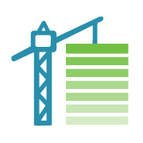# Math Fluency

Math fluency is one component of rigor as defined by the Common Core State Standards, where a student is developing conceptual understanding, procedural fluency, and application in equal intensity.

The following are the key shifts called for by the Common Core:

1. Focus strongly where the Standards focus
3. Rigor: In major topics, pursue conceptual understanding, procedural skill and fluency, and application with equal intensityFluency does not require using one particular method over another. Rather, students should be able to work confidently and efficiently. Fluent in the Standards means “fast and accurate.” This is similar to when we say that somebody is fluent in a foreign language: when you’re fluent, you flow. Fluent isn’t halting, stumbling, or reversing oneself.

However, speed and memorizing should not be the emphasis when we develop fluency. For example, a fluent thinker can multiply 24 times another number by decomposing the 24 -- first multiplying that number by 20, then by 4, and then adding these partial products. This is not a traditional algorithm, but it does demonstrate flexibility and fluency.

One of the 8 Teaching Practices named in the NCTM Principles to Action isBuild Procedural Fluency from Conceptual Understanding: Effective teaching of mathematics builds fluency with procedures on a foundation of conceptual understanding so that students, over time, become skillful in using procedures flexibly as they solve contextual and mathematical problems.

For example, this Kindergarten standard, K.OA.4, says:
For any number from 1 to 9, find the number that makes 10 when added to the given number, e.g., by using objects or drawings, and record the answer with a drawing or equation.

While this 1st grade standard, 1.OA.6, says:
... demonstrate fluency for addition and subtraction within 10

If a first grader is struggling with fluency for addition and subtraction within 10, support should focus on finding combinations of addends as described in the Kindergarten standard.

Fluency is developed via routines such as Math Talks and counting routines.

### This table summarizes the required fluency in grades K–6:

 K K.OA.5 Add/subtract within 5 1 1.OA.6 Add/subtract within 10 2 2.OA.2 ​2.NBT.5 Add/subtract within 20 (mental) Add/subtract within 100 (paper & pencil) 3 3.OA.7 ​3.NBT.2 Multiply/divide within 100 ​Add/subtract within 1000 4 4.NBT.4 Add/subtract within 1,000,000 5 5.NBT.5 Multi-digit multiplication 6 6.NS.2 & 3 Multi-digit division   Multi-digit decimal operations